Pointer是指针的英文单词

# 变量的地址和指针

int i,j,k;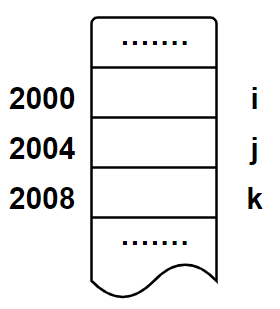i = 10;
j = 20;


k = i + j;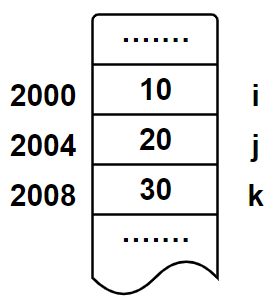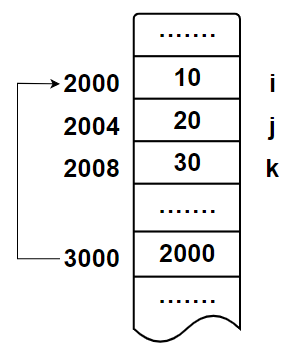# 指针变量的定义

//语法格式

//代码示例
int *a;	//声明了整型指针变量


1. 指针变量名前面的符号*在声明/定义时不可以省略，它是把其后变量声明为指针类型的标志。

2. 其他类型的变量允许和指针变量在同一个语句中定义。例如：

int a,b,*p;

3. 指针变量定义中的数据类型是指针指向的目标数据类型，而不是指针变量的数据类型。指针变量的数据类型由*声明为指针类型

# 指针运算

## 取地址运算符

&运算符是取地址运算符，它是单目运算符，其功能是返回其后所跟操作数的地址，其结合性为从右向左，例如：

int i=10,*p;
p=&i;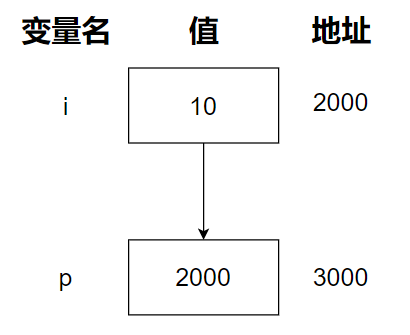## 指针运算符

*运算符是指针运算符，也称为间接运算符，它也是单目运算符。其功能是取该指针变量所指向的存储单元的值。代码示例：

int x=10,*p,y;	//声明和定义x=10，指针类型p,和整型变量y
p=&x;	//指针类型p取得x变量的地址
y=*p;	//将指针类型p指向的地址的值，即10赋值给y变量


## 赋值运算

### 指针变量的初始化

数据类型 * 指针变量名=地址1;


//取一般变量地址
int i;
int *p=&i;

//字符数组
char a;
int *p=a;


1. 不能用未声明的变量给指针变量赋初值，代码示例：

int *p = &i;
int i;

2. 当用一个变量地址为指针变量赋值时，该变量的数据类型必须与指针变量指向的数据类型一致。

3. 除0之外，一般不把其他整数作为初值赋给指针变量。程序运行期间，变量的地址是由计算机分配的，当用一个整数作为指针变量赋初值后，可能会造成难以预料的后果。当用0对指针赋初值时，系统会将该指针变量初始化为一个空指针，不指向任何对象。

### 使用赋值语句赋值

//语法格式

//代码示例
int *p,i;
p=&i;


int a=10,b=20,*p;
p=&a;
p=&b;


数据类型 *+变量名表示指针类型，*+变量名表示取指针的值

## 空指针与void指针

### 空指针

• 赋0值，这是唯一的允许不经转换就赋予指针的数值。

• NULL值，NULL值等于0，即两者等价，例如：

int *p;
p=0;
//或者
p=NULL;


### void指针

C语言规定，指针变量可以定义为void类型，例如：

void *p;


1. 任何指针都可以赋值给void指针

2. void指针赋值给其他类型指针需要进行类型转换。例如：

int *t=(int *)p;	//需要强制类型转换

3. void指针不能参与指针运算，除非进行转换。例如：

int main(){
void *c;
c++;	//编译出错，原因是不知道c的指向的类型
return 0;
}


# 指针与函数

## 指针作为函数参数

【实例】编写swap函数，实现交换两个整型变量的值。

【代码示例】

#include <stdio.h>
void Swap(int, int);	//函数原型声明
int main(){
int a = 1, b = 2;
printf("主函数交换前a的值为%d,b的值为%d\n", a, b);
Swap(a, b);	//交换函数
printf("主函数交换后a的值为%d,b的值为%d\n", a, b);
return 0;
}
void Swap(int a,int b){
int x;
x = a;
a = b;
b = x;
printf("交换后a的值为%d,b的值为%d\n", a, b);	//输出交换后的值
}


【输出】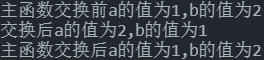【说明】从输出结果来看就可以知晓，对于调用函数来说，是实现了数的交换，但是只是形参的交换，而实参a,b并没有发生交换。这与我们想要的结果相差甚远，怎么办？

【实例】以指针为参数的交换swap函数，实现两数的交换。

【代码示例】

#include <stdio.h>
void Swap(int *, int *);
int main(){
int a = 1, b = 2;
printf("主函数交换前a的值为%d,b的值为%d\n", a, b);
Swap(&a, &b);
printf("主函数交换后a的值为%d,b的值为%d\n", a, b);
return 0;
}
void Swap(int *a,int *b){
int x;
x = *a;
*a = *b;	//获取传入值的存储空间并赋值
*b = x;
printf("交换后a的值为%d,b的值为%d\n", *a, *b);
}


【输出】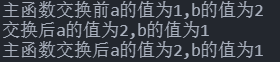#include <stdio.h>
void Swap(int *, int *);
int main(){
int a = 1, b = 2;
printf("主函数交换前a的值为%d,b的值为%d\n", a, b);
Swap(&a, &b);
printf("主函数交换后a的值为%d,b的值为%d\n", a, b);
return 0;
}
void Swap(int *a,int *b){
int *x;
x = a;
a = b;
b = x;
printf("交换后a的值为%d,b的值为%d\n", *a, *b);
}


【输出】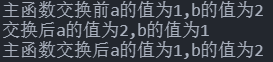“指针变量所指向单元的存储空间的值”和“指针变量存储的地址值是根本不同的。前者指的是指针指向单元的值，而后者指的是指针变量的值。

## 指针作为函数的返回值

数据类型 * 函数名(形参列表){
函数体;
}


【实例】输入若干数值，判断并获取最大值，且值的范围在$100$~$0$之间。

【代码示例】

#include <stdio.h>
int *Max();	//函数原型声明
int main(){
int *max;
max = Max();	//返回指针赋值
printf("最大值为：%d", *max);
return 0;
}
int * Max(){
static int max;	//静态局部变量，防止方法执行完毕内存被释放，值不存在
int n;
scanf("%d", &n);
while (n<100 && n>0)
{
if(n>max){
max = n;
}
scanf("%d", &n);
}
return &max;	//返回指针值，即地址
}


【输出】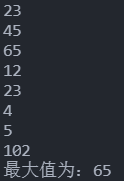## 函数指针

类型标识符 (*指针变量名)();


int *P();	//函数返回值类型为指针的函数，该指针指向整型
int (*p)();	//指向函数的指针，该函数返回值类型为整型


//语法格式

//代码示例
int Fun();	//函数原型声明
int (*F)();	//指向函数的指针声明
F=Fun;	//指向函数指针的地址指向Fun函数


【实例】使用函数指针，计算两个数的和

【代码示例】

#include <stdio.h>
int Plus(int,int);
int main(){
int (*P)(int,int); //声明函数指针
P = Plus;	//指针指向函数
printf("俩者的和为:%d",P(3,2));
return 0;
}
int Plus(int a,int b){
return a + b;
}


【输出】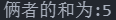【实例】关于函数指针的一些用法

【代码示例】

#include <stdio.h>
int Plus(int,int);  //加法函数原型声明
int Sub(int, int);  //减法函数原型声明
int Mul(int, int);  //乘法函数原型声明
int Fun(int, int, int (*P)(int, int));  //自定义函数原型声明
int main(){
printf("俩者的和为:%d\n",Fun(3,2,Plus));
printf("俩者的差为:%d\n",Fun(3,2,Sub));
printf("俩者的积为:%d\n",Fun(3,2,Mul));
return 0;
}
int Fun(int a,int b,int (*P)(int,int)){
return P(a, b); //自定义函数调用函数指针
}
int Plus(int a,int b){  //加法函数
return a + b;
}
int Sub(int a,int b){   //减法函数
return a - b;
}
int Mul(int a,int b){   //乘法函数
return a * b;
}


【输出】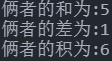# 指针与数组

## 一维数组的指针表示

### 定义指向一维数组的指针变量

int a={1,2,3},x;
x=a;


int a={1,2,3};
int *c=&a;	//获取指定元素的地址指针


int a={1,2,3};
int *p=a;
printf("%d",*p);


### 通过指针引用数组元素

C语言规定：如果指针变量p已指向数组中的一个元素，这p+1指向同一数组中的下一个元素

//常规方式
int a={1,2};
int c=a;

//指针方式
int *p,a={1,2,3};	//声明指针p和数组
p=a;	//指针指向数组首地址
for (int a = 0; a < 3; a++)
{
printf("%d\n",*p++);	//输出指针指向数值
}


### 数组中的指针运算

1. 加减算术运算

对于指向数组的指针变量，可以加上或者减去一个整数n。设p是指向数组a的指针变量，则p+np-np++p--等运算都是合法的。指针变量加减整数的意义是将指针指向的位置向前或者向后移动n个位置，这里加减的单位不是以字节为单位，而是以指向的数据类型所占用的字节数为单位。

【实例】指针算术运算示例

【代码示例】

#include <stdio.h>
int main(){
int a = {1, 2, 3, 4, 5}, *p, y = 30;
for (int i = 0; i < 5; i++)
{
printf("a[%d]=%d    ", i, a[i]);    //常规方法遍历输出数组元素
}
p = a;  //指针指向数组首地址
y = *++p;   //变量值赋值为指针向后移动一位的值
printf("\ny=%d  ", y);
printf("指针值为：%d", *p);
return 0;
}


【输出】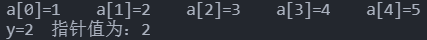2. 两个指针变量之间的运算

两个指针变量之间的加减运算是不合法的，也是无意义的，但是指针变量之间可以进行关系运算

假设指针p和指针q指向同一数组的元素，那么：

• p<q：当p所指的元素在q所指的前面时，表达式值为1；反之则为0；
• p>q：当p所指的元素在q所指的后面时，表达式值为1；反之则为0；
• p==q：当pq指向同一元素时，表达式值为1；反之则为0；
• p!=q：当pq不指向同一元素时，表达式值为1；反之则为0；

指针变量还可以与0或者NULL比较

p为指针变量，则p==0或者p==NULL表面是空指针，它不指向任何变量；p!=0或者p!=NULL表示p指针不是空指针。

【实例】指针变量间的关系运算

【代码示例】

#include <stdio.h>
int main(){
int a = {1, 2, 3, 4, 5}, *y, *x;
x = a;
y = a;
if(x<y){
printf("x<p\n");
printf("地址为：%p 值为：%d\n", x,*x);
printf("地址为：%p 值为：%d", y,*y);
}else{
printf("x>p\n");
printf("地址为：%p 值为：%d\n", x,*x);
printf("地址为：%p 值为：%d", y,*y);
}
if(y==0){
printf("\ny指针是空指针");
}else{
printf("\ny指针不是空指针");
}
return 0;
}


【输出】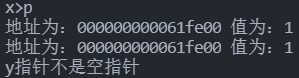## 二维数组的指针表示

### 用二维数组名表示数组元素

int a[M][N];


int a,*p;
p=a;	//指针变量指向数组a的第一个元素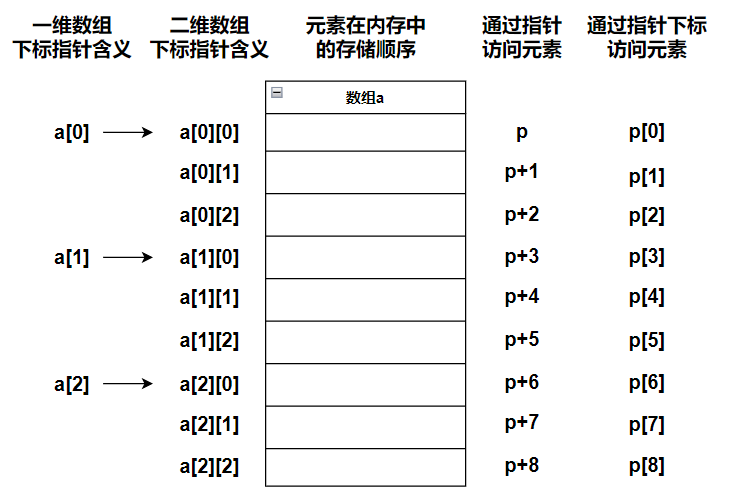//*(a+i) 取出指向i层首地址的地址
//*(a+i)+j 将该地址在该层移动j个单位，即指向第i层的第j个元素
//*(*(a+i)+j) 取出指针指向地址的值，即取出第i层的第j个元素的值
*(*(a+i)+j);


【实例】关于数组指针相关问题理解

【代码示例】

#include <stdio.h>
int main(){
//定义了一维数组c,二维数组a,三维数组x
int a = {{1, 2}, {3, 4}},c={5,6},x={{{1,1},{2,2}},{{3,3},{4,4}}};
//获取一维数组首地址的值，因为数组名是常量指针，默认指向首元素地址
printf("一维数组：%d\n", *c);
//获取二维数组第一层的第二个元素
//*a指的是首层地址，+1是在该层的基础上移动一个单位，即首层第二个元素
printf("二维数组：%d\n", *(*a+1));
//a以及指明了数组第二层首元素的地址，*取其值
printf("二维数组：%d\n", *a);
//*x取首层元素首地址，**x取首层元素首层元素的地址，***x取首层元素首层元素首层元素的值
//因为是三维数组，所以嵌套三层才能确定具体元素的地址
printf("三维数组：%d\n", ***x);
return 0;
}


【输出】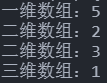### 用指针表示二维数组元素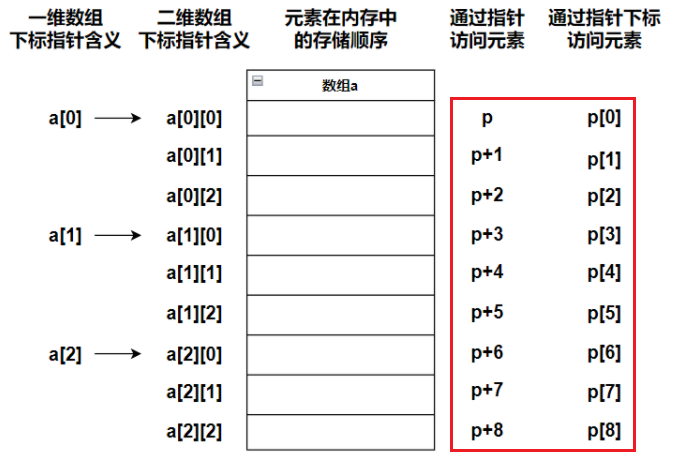$$p+M \times N +y$$

int a[M][N];
int *p=a;


a*a指的是同一个地址，即首层首元素地址。

【实例】利用指针输出二维数组元素值

【代码示例】

#include <stdio.h>
int main(){
int a = {{1, 2}, {3, 4}},*p;
for (int i = 0; i < 2; i++)
{
p = a[i];
for (int j = 0; j < 2; j++)
{
printf("第%d层第%d个元素的值为：%d\n", i+1, j+1, *(p + j));
}
}

return 0;
}


【输出】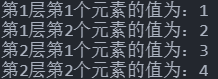类型关键字 (*行指针名)[M]


int (*p)[M];


【实例】使用行指针输出二维数组中全部的元素值

【代码示例】

#include <stdio.h>
int main(){
int(*p),a={{1,2,3},{4,5,6}};	//声明行指针
p = a;	//行指针指向首行数组首地址
for (int i = 0; i < 3; i++)
{
printf("%d", *(*p+i));
}
return 0;
}


p层加减操作是改变层数的指向，对*p的操作是改变的元素的指向

【关于指针指向多维数组的个人看法】

C语言底层就是将多维数组，例如二维数组，看成两个一维数组，这就意味着，对于二维数组a，之前说过数组是一个常量指针，那么a是指向第一个一维数组的指针，也就是说a存储的是一个地址，这也就是为什么如果使用普通指针指向二维数组a，例如：*a获取的只是第一个一维数组的地址，而**a才是获取第一个一维数组第一个元素的首元素的值。

## 指针与字符串

C语言没有字符串变量，对字符串的访问有两种方法。

1. 使用字符数组来存放一个字符串，然后采用字符数组来完成操作。例如：

char a="hello world";
//输出
printf("%s",a);

2. 使用字符指针指向一个字符串

如果把字符数组的首地址赋给一个指针变量，那么这个指针变量指向这个数组，就可以对齐完成数组操作。

//使用字符串常量对字符指针进行初始化，此时指针变量指向字符串的首地址。
char *str="hello world";


【实例】编写程序完成字符串的输出

#include <stdio.h>
int main(){
char *str = "hello world";	//初始化字符串指针
printf("第三个字符是：%c\n", str);
for (int i = 0; i < 12; i++)
{
printf("%c", *str++);
}
return 0;
}


【输出】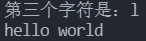# 指针数组和指向指针的指针

## 指针数组

1. 指针数组的定义

如果数组中每个元素的数据类型为指针类型，则这种数组称为指针数组，它是指针的集合。语法格式如下：

//语法格式
类型 * 数组名[常量表达式];
//代码示例
int *p;	//表示定义一个由10个指针变量构成的指针数组。

2. 指针数组在字符串中的使用

指针数组常用来表示一组字符串，这时指针数组的每个元素被赋予每个字符串的首地址。代码示例：

char *str={"Sunday","Monday","Tuesday","Wednesday","Thursday","Friday","Saturday"};


当然也可以使用一个二维数组来表示上面的信息，代码示例：

char week={"Sunday","Monday","Tuesday","Wednesday","Thursday","Friday","Saturday"};


它们在内存的存储结结构如下所示：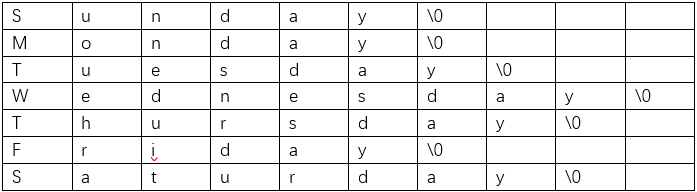该数组一共占用70个字节。

如果采用指针数组来表示，由于指针数组的每个元素都是指针，因此指向每个元素的首地址，通过首地址访问该字符串。相对于二维数组可以节省内存空间。如下：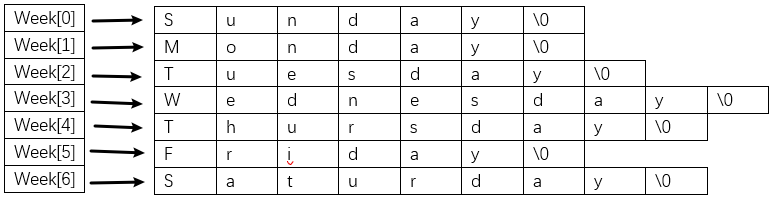【实例】编写程序，用星期的英文名来初始化字符指针数组，输入整数，当数为0~6时，输出对应星期的英文名，否则显示错误信息。

【代码示例】

#include <stdio.h>
void PutOut(char *p[], int value);
int main(){
char *weekday = {"Sunday", "Monday", "Tuesday", "Wednesday", "Thursday", "Friday", "Saturday"};
int value;
printf("请输入数值");
scanf("%d", &value);
PutOut(weekday,value);
return 0;
}
void PutOut(char *p[],int value){
if(value>=0 && value<=6){
printf("%s", p[value]);
}else{
printf("输出格式不正确");
}
}


【输出】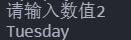## 指向指针的指针

//语法格式

//代码示例
int ** p;


int x=2;	//初始化整型变量x=2
int *p;		//声明指针p
int **q;	//声明二级指针q
p=&x;	//定义指针p指向变量x
q=&p;	//定义二级指针q指向指针p
printf("%d",**q);	//输出二级指针所指向指针指向的值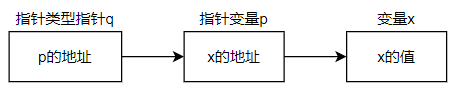# End

Q.E.D.

••